sk|cz|

1.Solve in real numbers: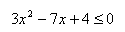Solution: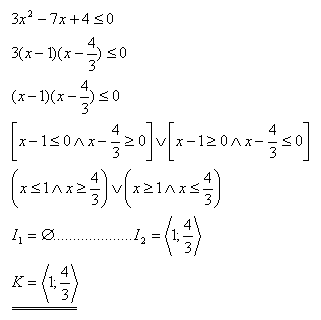2.Solve in real numbers: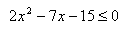Solution: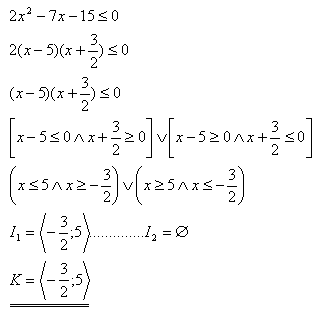3.Solve in real numbers: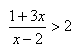Solution: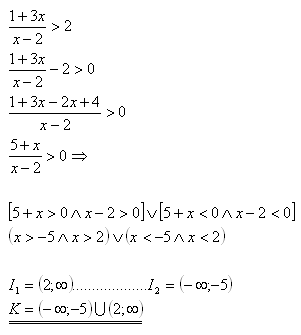4.Find all „a“ so that the following equation would have a possitive solution: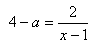Solution: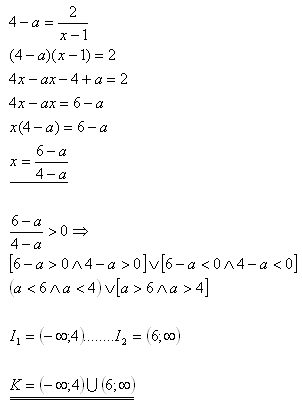5.Find all „m“ real numbers so that the following equation would have a root greater or equal 4: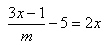Solution: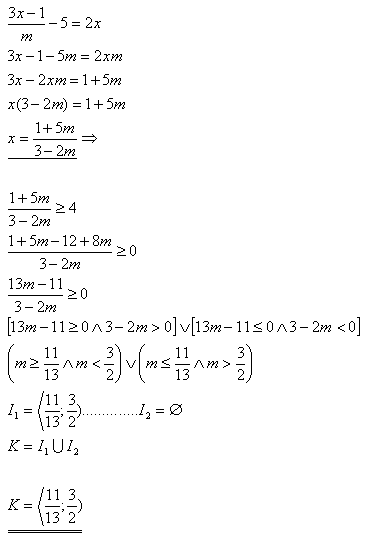6.For which „a“ would both the roots of the set of equations be negative ( x<0; y<0 ) ?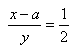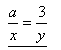Solution: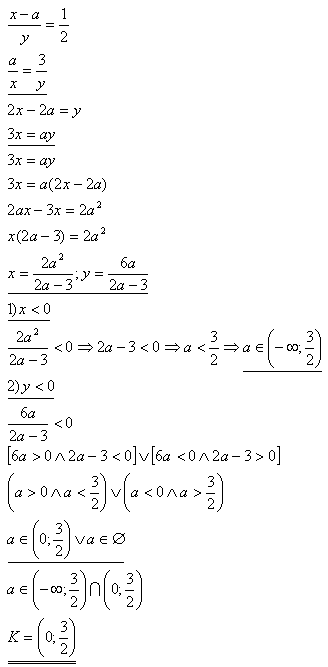7. Solve in negative integers: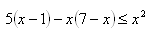Solution: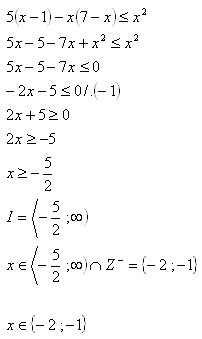8.Solve in real numbers: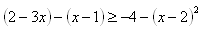Solution: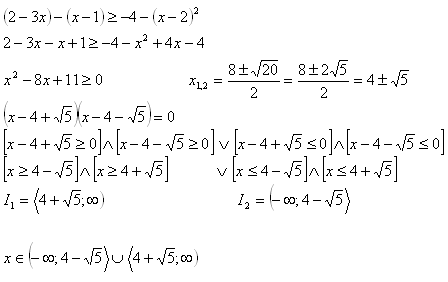9.Solve in real numbers: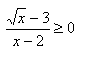Solution: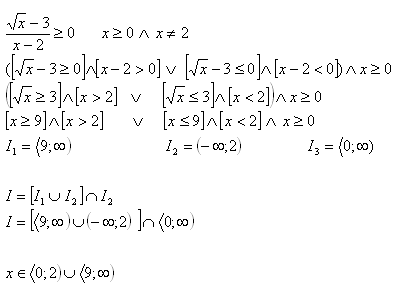10. Which „x“ fits the inequality: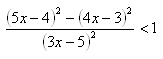Solution: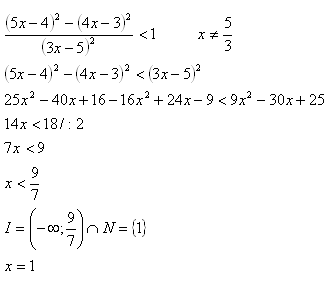For the inequality, x = 1.